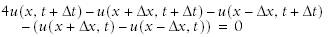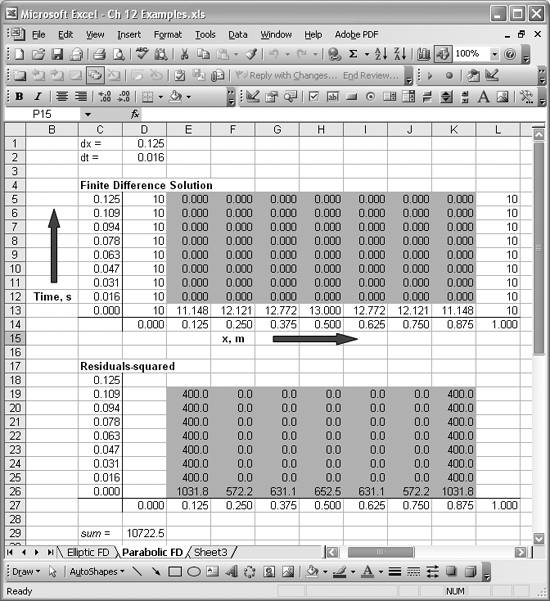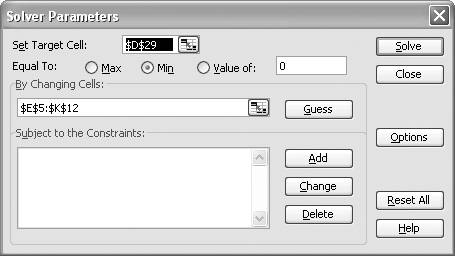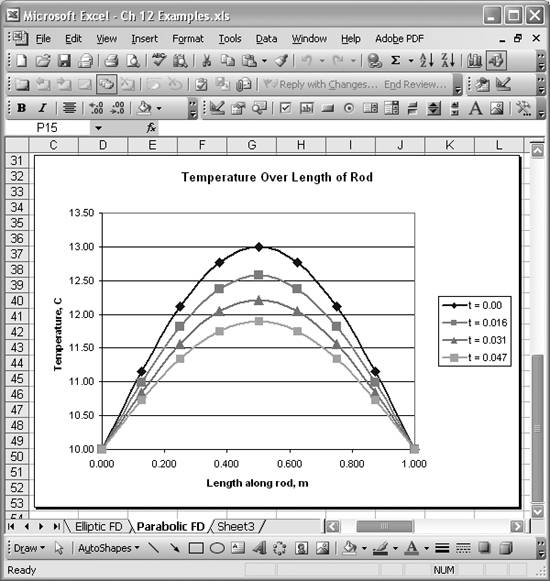# Solving Initial Value Problems

Problem

You're dealing with a parabolic or hyperbolic problem and have developed the finite difference equations for the problem, which includes both discretized spatial and time variables. Now you have to solve these equations.

Solution

Use Excel and Solver to solve the finite difference equations in a manner similar to that discussed in Recipe 12.2.

Discussion

In the previous recipe, I showed you how to leverage Solver to solve the finite difference equations, arriving at a steady state solution to an elliptic-type boundary value problem. You can apply the same techniques to solve time-dependent parabolic or hyperbolic types of problems.

Consider the time-dependent one -dimensional heat equation:This is a parabolic equation, which can be used to model the time-dependent heat conduction in a metal rod, for example, subject to some prescribed initial temperature distribution and boundary conditions at the ends of the rod.

Let's assume we have a 1m rod that's insulated all around except at the ends. The ends of the rod are maintained at a constant 10°C and the initial temperature distribution along the length of the rod (at time t = 0) is as shown in Figure 12-7, row 13. Further assume that c2 equals 1. Now we can use the finite difference method to solve for the temperature distribution along the length of the rod over time.

Erwin Kreyszig solves a similar problem in Advanced Engineering Mathematics.[*] Kreyszig uses the Crank-Nicolson finite difference method, where the following finite difference equation applies at each computational node in the finite difference grid:

[*] Erwin Kreyszig, Advanced Engineering Mathematics, 6th ed. (New York: Wiley, 1988).In this method, both spatial and time variables are discretized. You can set up a finite difference grid similar to that shown in Figure 12-2, where instead of the vertical axis representing the y-dimension, the vertical axis will now represent time. The horizontal axis still represents the x-dimensionthe distance along the rod's length in this case. The finite difference equation shown here assumes that the time step size, Dt, is related to the x step size, Dx, such that Dt =Dx2.

You can solve this problem in a manner similar to that discussed in Recipe 12.2, where I showed you how to solve a boundary value problem using Solver. Figure 12-7 shows the spreadsheet I set up to solve this initial value problem.

The upper table, Finite Difference Solution, initially contains only specified boundary and initial values (nonshaded cells) and initial guesses for the unknown temperature values along the length of the rod (shaded cells). We're going to let Solver alter the values in the shaded cells in the upper table to find a solution.

The shaded cells in the lower table, Residuals-squared, contain the finite difference equation shown earlier for each node. Actually, the square of the lefthand side, the residual, is computed; the idea is to minimize these residuals as discussed earlier. For example, the formula in cell H22 is =(4*H8-I8-G8-I9-G9)^2. All the other cell formulas are similar. Notice that these formulas refer to values contained in the upper table.

Cell D29 contains the sum of all these residual cells. We're going to have Solver attempt to minimize the value computed in cell D29. The Solver model I used for this example is shown in Figure 12-8.

Figure 12-7. Spreadsheet for solving initial value problemFigure 12-8. Solver model for initial value problemI set the target cell to D29 so as to minimize the sum of squared residuals. The cells to change are all the shaded cells in the upper table of Figure 12-7. The corresponding cell range is E5 to K12. Pressing the Solve button sets Solver going on its search for a solution. After a few seconds, Solver presents the results shown in Figure 12-9.

Figure 12-9. Final solution to initial value problemAs you can see, all the residuals are 0 and the upper table now contains the solution to this initial value problem. Figure 12-10 shows a plot of these results.

As I mentioned in the previous recipe, the ability to quickly visualize your results in Excel without having to use a separate visualization tool is another compelling reason to use Excel for this sort of problem when possible. Refer to Chapter 4 to learn more about creating charts in Excel.

Figure 12-10. Plot of initial value problem resultsExcel Scientific and Engineering Cookbook (Cookbooks (OReilly))
ISBN: 0596008791
EAN: 2147483647
Year: N/A
Pages: 206
Authors: David M Bourg

Similar book on Amazon